## RS Aggarwal Class 8 Solutions Chapter 1 Rational Numbers Ex 1E

These Solutions are part of RS Aggarwal Solutions Class 8. Here we have given RS Aggarwal Solutions Class 8 Chapter 1 Rational Numbers Ex 1E.

Other Exercises

Question 1.
Solution: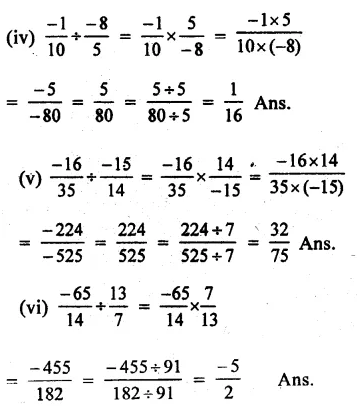Question 2.
Solution: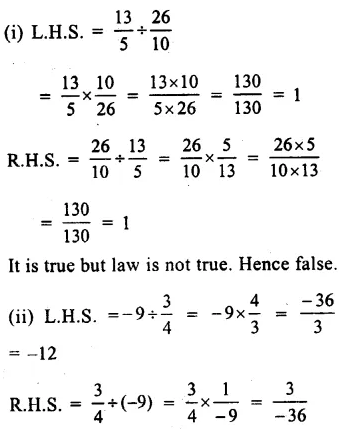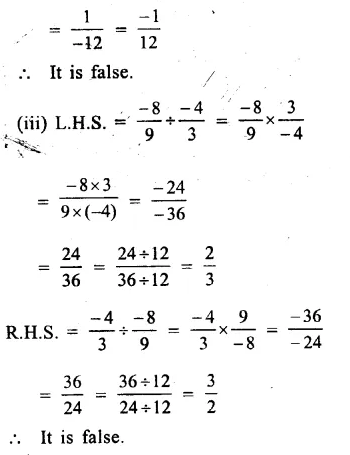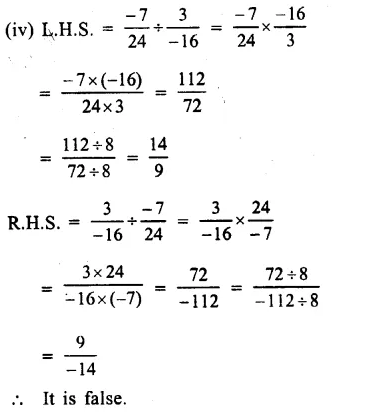Question 3.
Solution: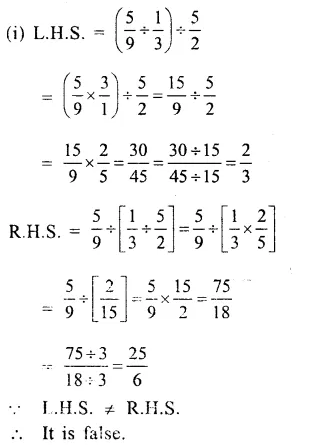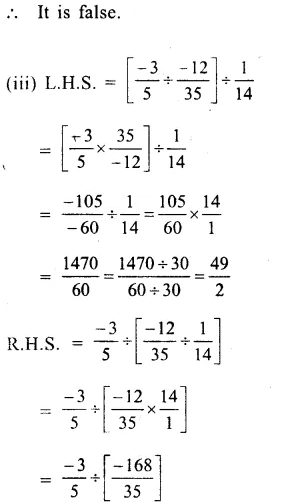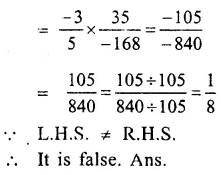Question 4.
Solution:
Product of two numbers = – 9
one number = – 12
Let second number = xQuestion 5.
Solution:
Product of two rational numbers = $$\\ \frac { -16}{ 9 }$$
One number = $$\\ \frac { -4 }{ 3 }$$Question 6.
Solution:
Let x be multiplied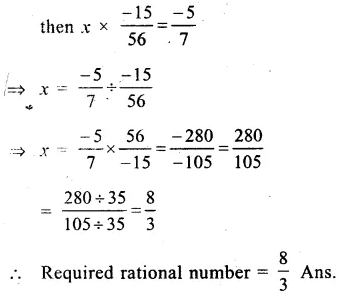Question 7.
Solution:
Let x be multiplied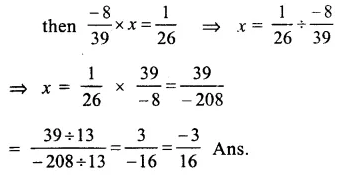Question 8.
Solution:
Let required number = x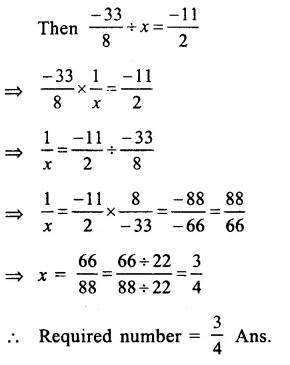Question 9.
Solution:
sum of $$\\ \frac { 13 }{ 5 }$$ and $$\\ \frac { -12 }{ 7 }$$
= $$\\ \frac { 13 }{ 5 }$$ + $$\\ \frac { -12 }{ 7 }$$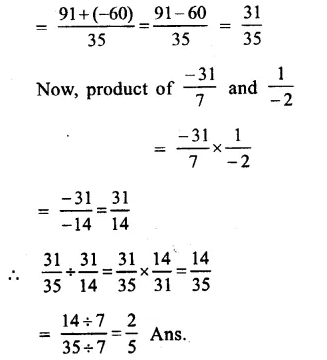Question 10.
Solution: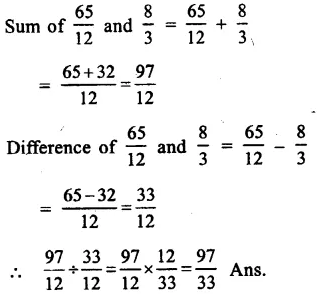Question 11.
Solution: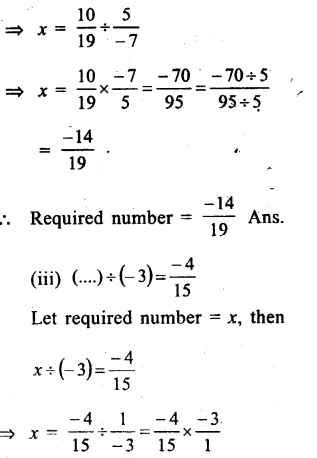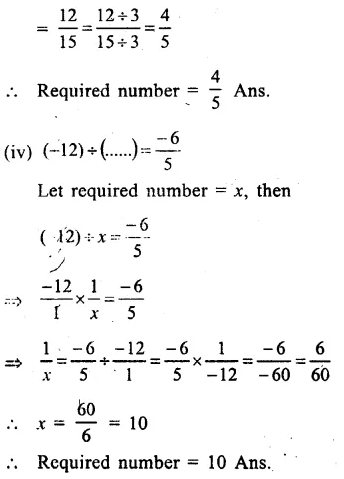Question 12.
Solution:
(i) No, not always closed under division.
(ii) No, not always commutative.
(iii) No, not always associative.
(iv) No. It is not possible to divide any number by zero.

Hope given RS Aggarwal Solutions Class 8 Chapter 1 Rational Numbers Ex 1E are helpful to complete your math homework.

If you have any doubts, please comment below. Learn Insta try to provide online math tutoring for you.